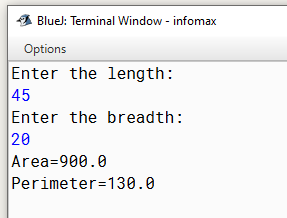# Java program to input the length and breadth of a rectangle and find its area and perimeter

Write a program to input the length and breadth of a rectangle and find its area and perimeter.

Note:

`Solution`

``````import java.util.*;
class Demo
{
public static void main(String arr[])
{
Scanner sc=new Scanner(System.in);
float l,b,a,p;
System.out.println("Enter the length:");
l=sc.nextFloat();
b=sc.nextFloat();
a=l*b;
p=2*(l+b);
System.out.println("Area="+a);
System.out.println("Perimeter="+p);
}
}
``````
`Output`Write a program to input the length and breadth of a rectangle and find its area and perimeter.

`Solution`

``````import java.util.*;
class AreaPerimeter
{
public static void main(String arr[])
{
Scanner sc=new Scanner(System.in);
float l,b,a,p;
System.out.println(“Enter the length:”);
l=sc.nextFloat();
b=sc.nextFloat();
a=l*b;
p=2*(l+b);
System.out.println(“Area=”+a);
System.out.println(“Perimeter=”+p);
}
}``````
`Output`

Write a program to input the length and breadth of a rectangle and find its area and perimeter.